# swt2

Discrete stationary 2-D wavelet transform

## Syntax

``[A,H,V,D] = swt2(X,N,wname)``
``[A,H,V,D] = swt2(X,N,LoD,HiD)``
``swc = swt2(___)``

## Description

example

````[A,H,V,D] = swt2(X,N,wname)` returns the approximation coefficients `A` and the horizontal, vertical, and diagonal detail coefficients `H`, `V`, and `D`, respectively, of the stationary 2-D wavelet decomposition of the image `X` at level `N` using the wavelet `wname`. Note`swt2` is uses periodic extension.`swt2` uses double-precision arithmetic internally and returns double-precision coefficient matrices. `swt2` warns if there is a loss of precision when converting to double. ```
````[A,H,V,D] = swt2(X,N,LoD,HiD)` uses the specified lowpass and highpass wavelet decomposition filters `LoD` and `HiD`, respectively.```
````swc = swt2(___)` returns the approximation and detail coefficients in `swc`.```

## Examples

collapse all

```load woman imagesc(X) colormap(map) title('Original')```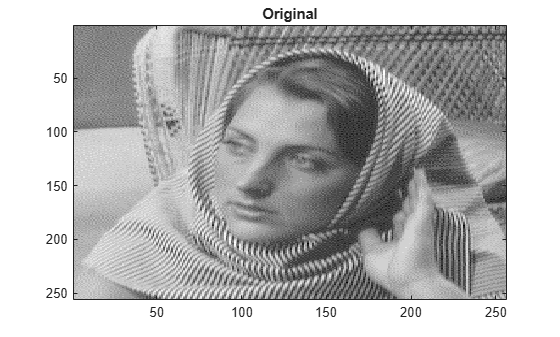Perform the stationary wavelet decomposition of the image at level 2 using `db6`.

`[ca,chd,cvd,cdd] = swt2(X,2,'db6');`

Extract the level 1 and level 2 approximation and detail coefficients from the decomposition.

```A1 = wcodemat(ca(:,:,1),255); H1 = wcodemat(chd(:,:,1),255); V1 = wcodemat(cvd(:,:,1),255); D1 = wcodemat(cdd(:,:,1),255); A2 = wcodemat(ca(:,:,2),255); H2 = wcodemat(chd(:,:,2),255); V2 = wcodemat(cvd(:,:,2),255); D2 = wcodemat(cdd(:,:,2),255);```

Display the approximation and detail coefficients from the two levels.

```subplot(2,2,1) imagesc(A1) title('Approximation Coef. of Level 1') subplot(2,2,2) imagesc(H1) title('Horizontal Detail Coef. of Level 1') subplot(2,2,3) imagesc(V1) title('Vertical Detail Coef. of Level 1') subplot(2,2,4) imagesc(D1) title('Diagonal Detail Coef. of Level 1')```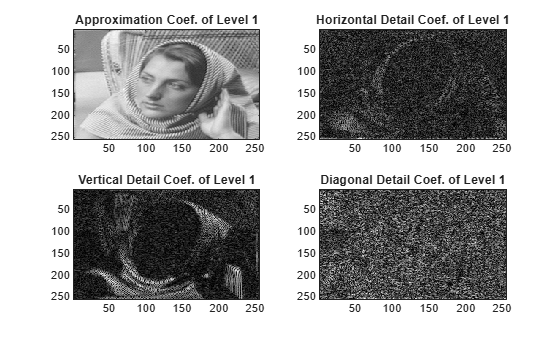```subplot(2,2,1) imagesc(A2) title('Approximation Coef. of Level 2') subplot(2,2,2) imagesc(H2) title('Horizontal Detail Coef. of Level 2') subplot(2,2,3) imagesc(V2) title('Vertical Detail Coef. of Level 2') subplot(2,2,4) imagesc(D2) title('Diagonal Detail Coef. of Level 2')```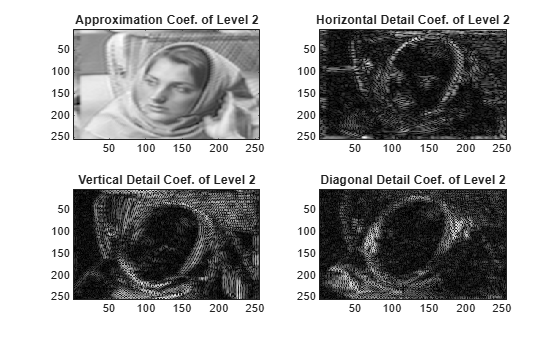This example shows how to obtain single-level and multilevel stationary wavelet decompositions of an RGB image.

Load and view an RGB image. The image is a 3-D array of type `uint8`. Since `swt2` requires that the first and second dimensions both be divisible by a power of 2, extract a portion of the image.

```imdata = imread('ngc6543a.jpg'); x = imdata(1:512,1:512,:); image(x) title('RGB Image')```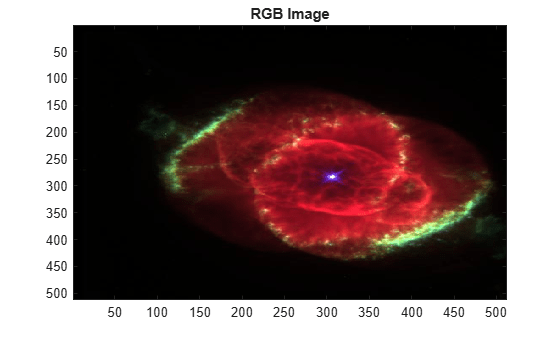Obtain the level 4 stationary wavelet decomposition of the image using the `db4` wavelet. Return the approximation coefficients. Note the dimensions of the coefficients array.

```[a,~,~,~] = swt2(x,4,'db4'); size(a)```
```ans = 1×4 512 512 3 4 ```

The coefficients are all of type `double`. In an RGB array of type `double`, each color component is a value between 0 and 1. Rescale the level 2 approximation coefficients to values between 0 and 1 and view the result.

```a2 = a(:,:,:,2); a2 = (a2-min(a2(:)))/(max(a2(:))-min(a2(:))); image(a2) title('Level 2 Approximation')```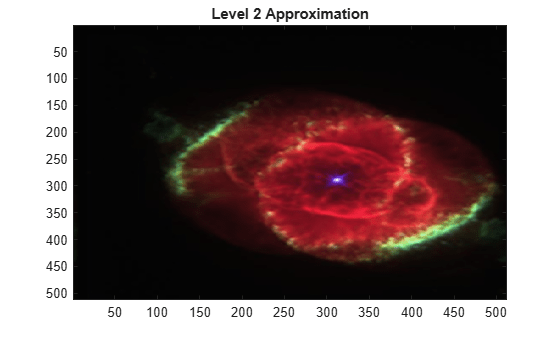Obtain the single-level stationary wavelet decomposition of the image using the `db4` wavelet. Return the approximation coefficients. In a single-level decomposition of an RGB image, the third dimension is singleton.

```[a,~,~,~] = swt2(x,1,'db4'); size(a)```
```ans = 1×4 512 512 1 3 ```

View the approximation coefficients. To prevent an error when using `image`, squeeze the approximation coefficients array to remove the singleton dimension.

```a2 = squeeze(a); a2 = (a2-min(a(:)))/(max(a(:))-min(a(:))); image(a2) title('Approximation')```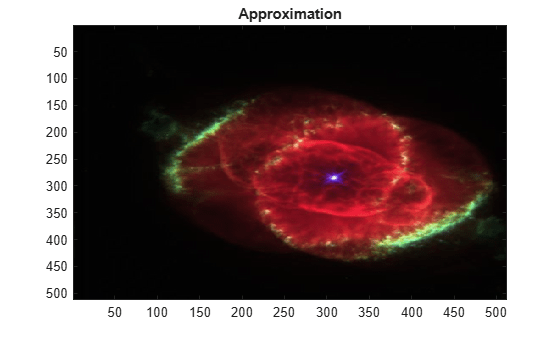## Input Arguments

collapse all

Input image, specified as a real-valued 2-D matrix or real-valued 3-D array. If `X` is 3-D, `X` is assumed to be an RGB image, also referred to as a truecolor image, and the third dimension of `X` must equal 3. For more information on truecolor images, see Image Types.

Data Types: `double`

Level of decomposition, specified as a positive integer. 2N must divide `size(X,1)` and `size(X,2)`. Use `wmaxlev` to determine the maximum level of decomposition.

Analyzing wavelet, specified as a character vector or string scalar. `swt2` supports only Type 1 (orthogonal) or Type 2 (biorthogonal) wavelets. See `wfilters` for a list of orthogonal and biorthogonal wavelets.

Wavelet decomposition filters, specified as a pair of even-length real-valued vectors. `LoD` is the lowpass decomposition filter, and `HiD` is the highpass decomposition filter. The lengths of `LoD` and `HiD` must be equal. See `wfilters` for additional information.

## Output Arguments

collapse all

Approximation coefficients, returned as a multidimensional array. The dimensions of `A` depend on the dimensions of the input `X` and the level of decomposition `N`.

• If `X` is m-by-n:

• If `N` is greater than 1, then `A` is m-by-n-by-`N`. For 1 ≤ i`N`, `A(:,:,i)` contains the approximation coefficients at level i.

• If `N` is equal to 1, then `A` is m-by-n.

• If `X` is m-by-n-by-3:

• If `N` is greater than 1, then `A` is m-by-n-by-3-by-`N`. For 1 ≤ i`N` and `j = 1, 2, 3`, `A(:,:,j,i)` contains approximation coefficients at level i.

• If `N` is equal to 1, then `A` is m-by-n-by-1-by-3. Since MATLAB® removes singleton last dimensions by default, the third dimension is singleton.

Data Types: `double`

Detail coefficients, returned as multidimensional arrays of equal size. `H`, `V`, and `D` contain the horizontal, vertical, and diagonal detail coefficients, respectively. The dimensions of the arrays depend on the dimensions of the input `X` and the level of decomposition `N`.

• If `X` is m-by-n:

• If `N` is greater than 1, the arrays are m-by-n-by-`N`. For 1 ≤ i`N`, `H(:,:,i)`, `V(:,:,i)`, and `D(:,:,i)` contain the detail coefficients at level i.

• If `N` is equal to 1, the arrays are m-by-n.

• If `X` is m-by-n-by-3:

• If `N` is greater than 1, the arrays are m-by-n-by-3-by-`N`. For 1 ≤ i`N` and `j = 1, 2, 3`, `H(:,:,j,i)`, `V(:,:,j,i)`, and `D(:,:,j,i)` contain the detail coefficients at level i.

• If `N` is equal to 1, the arrays are m-by-n-by-1-by-3. For `j = 1, 2, 3`, `H(:,:,1,j)`, `V(:,:,1,j)` and `D(:,:,1,j)` contain the detail coefficients. Since MATLAB removes singleton last dimensions by default, the third dimension is singleton.

Data Types: `double`

Stationary wavelet decomposition, returned as a multidimensional array. `swc` is the concatenation of the approximation coefficients `A` and detail coefficients `H`, `V`, and `D`.

• If `X` is m-by-n and `N` is greater than 1, then ```swc = cat(3,H,V,D,A(:,:,N))```.

• If `X` is m-by-n and `N` is equal to 1, then ```swc = cat(3,H,V,D,A)```.

• If `X` is m-by-n-by-3 and `N` is greater than 1, then ```swc = cat(4,H,V,D,A(:,:,:,N))```.

• If `X` is m-by-n-by-3 and `N` is equal to1, then ```swc = cat(4,H,V,D,A)```.

## Algorithms

collapse all

### 2-D Discrete Stationary Wavelet Transform

For images, a stationary wavelet transform (SWT) algorithm similar to the one-dimensional case is possible for two-dimensional wavelets and scaling functions obtained from one-dimensional functions by tensor product. This kind of two-dimensional SWT leads to a decomposition of approximation coefficients at level j into four components: the approximation at level j+1, and the details in three orientations (horizontal, vertical, and diagonal).

This chart describes the basic decomposition step for images.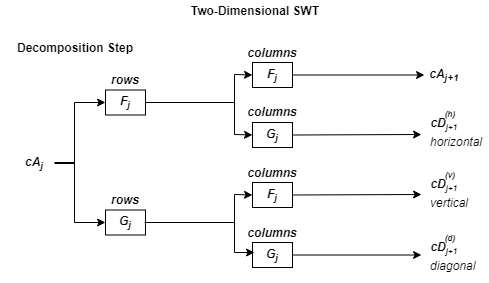where

•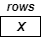— Convolve the rows of the entry with filter X.

•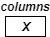— Convolve the columns of the entry with filter X.

Initialization

• cA0 = s

• F0 = `LoD`

• G0 = `HiD`

•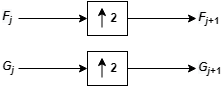where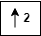denotes upsample.

Note that ```size(cAj) = size(cDj(h)) = size(cDj(v)) = size(cDj(d)) = s```, where s equals the size of the analyzed image.

### Truecolor Image Coefficient Arrays

To distinguish a single-level decomposition of a truecolor image from a multilevel decomposition of an indexed image, the approximation and detail coefficient arrays of truecolor images are 4-D arrays.

• If you perform a multilevel decomposition, the dimensions of `A`, `H`, `V`, and `D` are m-by-n-by-3-by-k, where k is the level of decomposition.

• If you perform a single-level decomposition, the dimensions of `A`, `H`, `V`, and `D` are m-by-n-by-1-by-3. Since MATLAB removes singleton last dimensions by default, the third dimension of the arrays is singleton.

 Nason, G. P., and B. W. Silverman. “The Stationary Wavelet Transform and Some Statistical Applications.” In Wavelets and Statistics, edited by Anestis Antoniadis and Georges Oppenheim, 103:281–99. New York, NY: Springer New York, 1995. https://doi.org/10.1007/978-1-4612-2544-7_17.

 Coifman, R. R., and D. L. Donoho. “Translation-Invariant De-Noising.” In Wavelets and Statistics, edited by Anestis Antoniadis and Georges Oppenheim, 103:125–50. New York, NY: Springer New York, 1995. https://doi.org/10.1007/978-1-4612-2544-7_9.

 Pesquet, J.-C., H. Krim, and H. Carfantan. “Time-Invariant Orthonormal Wavelet Representations.” IEEE Transactions on Signal Processing 44, no. 8 (August 1996): 1964–70. https://doi.org/10.1109/78.533717.